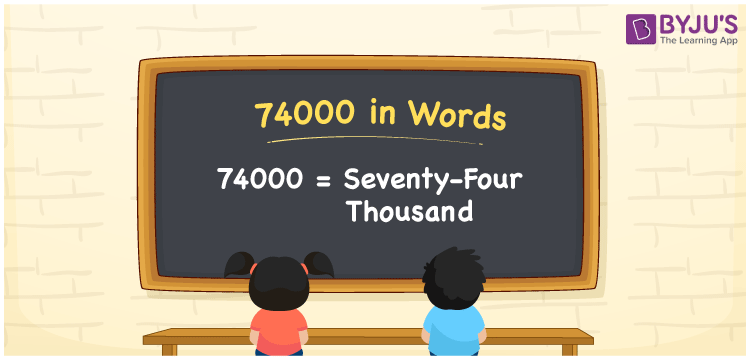# 74000 in Words

74000 in words is expressed as Seventy-Four Thousand. On a cheque, we can write Rs. 74000 as “Rupees Seventy-Four Thousand Only”. We know that 74000 is a cardinal number as it represents the value or quantity. Learn to write 74000 in words using a place value chart at BYJU’S.

 74000 in words Seventy-Four Thousand Seventy-Four Thousand in numerical form 74000

## 74000 in English Words

Generally, numbers in words are represented by the English alphabet. Therefore, 74000 in English is written as Seventy-Four Thousand.## How to Write 74000 in Words?

74000 can be written in words using a place value chart. 74000 is a five-digit number. Thus, let us make a place value chart up to five digits. Learn the position of each digit from the below chart.

 Ten Thousands Thousands Hundreds Tens Ones 7 4 0 0 0

Therefore, we can write the expanded form as:

7 x Ten Thousand + 4 x Thousand + 0 x Hundred + 0 × Ten + 0 × One

= 7 x 10000 + 4 x 1000 + 0 x 100 + 0 x 10 + 0 x 1

= 70000 + 4000 + 0 + 0 + 0

= 70000 + 4000

= 74000

= Seventy-Four Thousand

Hence, 74000 in words is written as Seventy-Four Thousand.

Interesting way of writing 74000 in words

7 = Seven

74 = Seventy-Four

740 = Seven Hundred and Forty

7400 = Seven Thousand Four Hundred

74000 = Seventy-Four Thousand

Thus, the word form of the number 74000 is Seventy-Four Thousand.

74000 is a natural number that is succeeded by 73999 and preceded by 74001

• 74000 in words – Seventy-Four Thousand
• Is 74000 an odd number? – No
• Is 74000 an even number? – Yes
• Is 74000 a perfect square number? – No
• Is 74000 a perfect cube number? – No
• Is 74000 a prime number? – No
• Is 74000 a composite number? – Yes

## Frequently Asked Questions on 74000 in Words

Q1

### Write 74000 in words.

74000 in words is written as Seventy-Four Thousand.
Q2

### What is the value of 68000 plus 6000 in words?

68000 + 6000 = 74000 Hence, the value of 68000 plus 6000 in words is Seventy-Four Thousand.
Q3

### 74000 is a prime number. True or False.

False. 74000 is not a prime number.